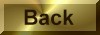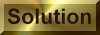Practice Problem 6

CalculateH° andS°   for the following reaction:

NH4NO3(s)  + H2O(lNH4+ (aq) + NO3- (aq)

Use the results of this calculation to determine the value ofGo for this reaction at 25o C, and explain why NH4NO3 spontaneously dissolves is water at room temperature.Ho = 28.05 kJSo = 108.7 J/KGo = -4.4 kJ